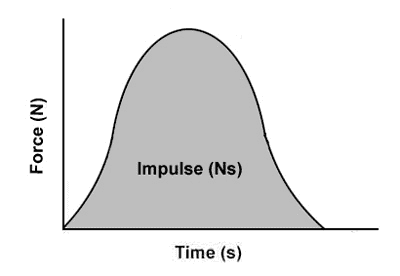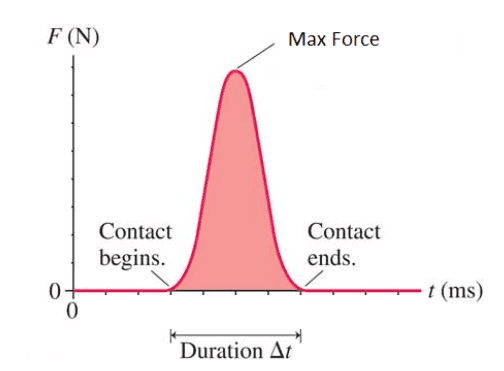# Using derivatives in physics

• NODARman
In summary, the conversation discusses calculating physics problems involving derivatives and real numbers. It mentions using the formula dP/dt = Fnet to calculate impulse, and the concept of taking a calculus course to better understand these calculations. It also mentions the importance of considering infinitesimal time intervals and the role of the Calculus in solving complex problems.f

#### NODARman

Homework Statement
.
Relevant Equations
.
Hi, I'm trying to calculate my own physics problem but didn't get it something.
When I'm trying to calculate the impulse of the object when it's hit by F=10N force in the smallest possible time, then should I write:
dP/dt = Fnet => dP = Fnet*dt ?

Another question: In general, if I calculate just a net force, we know that the derivative of the constant is zero. Then if dP is 10 N/s and t->0 therefore Fnet = 10/0 ?
What I don't get is how to calculate any physics formula which contains derivatives and real numbers.

I don’t think any collision actually lasts 0 seconds. A collision that lasts 0 seconds is a collision that doesn’t actually happen.

Also ##dP## would have units of ##N \cdot s## not ##\frac{N}{s}##.

•topsquark, malawi_glenn and NODARmanThese might help

and if ##\dfrac{\mathrm{d}p}{\mathrm{d}t} = F(t)## then ## \displaystyle \Delta p = \int_{t_1}^{t_2}F(t) \mathrm{d}t##
Now one can define the average constant force of impulse as ##<F> = \Delta p / \Delta t = \Delta p / (t_2 - t_1) ##
i.e. ## \displaystyle <F> = \dfrac{1}{t_2 - t_1} \int_{t_1}^{t_2}F(t) \mathrm{d}t## which you might remember from calculus class.

Last edited:
•topsquark, NODARman, PeroK and 1 other person
What I don't get is how to calculate any physics formula which contains derivatives and real numbers.
You could take a calculus course?

•FactChecker, topsquark and malawi_glenn
When I'm trying to calculate the impulse of the object when it's hit by F=10N force in the smallest possible time
There's no such thing as the smallest amount of time. Unless you mean zero, in which case no collision occurs. The collision must last for some nonzero amount of time, however small.

•NODARman
Homework Statement:: .
Relevant Equations:: .

What I don't get is how to calculate any physics formula which contains derivatives and real numbers.
You should not feel bad, it took a fellow named Newton to figure out how to deal with instantaneous change.
To any degree of approximation for a well behaved force, slicing time into finite intervals Δt, assuming the force is constant over that interval and summing will get you a numerical result to nearly any level of accuracy required.
But doing the clever stuff like calculating exact orbits for all time requires a leap of intuition. What happens to this rate of change as I slice time into infinitesimal pieces (dt) ?? The answer is called The Calculus and it is a touchstone for human ingenuity. It is not easy.

•NODARman, bob012345 and PhDeezNutz